Beams Index

Elastic Bending Theory

Introduction.... Superposition.... Nomenclature.... Simple Bending.... Deflection.... Area Moment Method.... Composite Beams....

 Introduction The stress, strain, dimension, curvature, elasticity, are all related, under certain assumption, by the theory of simple bending.   This theory relates to beam flexure resulting from couples applied to the beam without consideration of the shearing forces. Superposition Principle The superposition principle is one of the most important tools for solving beam loading problems allowing simplification of very complicated design problems.. For beams subjected to several loads of different types the resulting shear force, bending moment, slope and deflection can be found at any location by summing the effects due to each load acting separately to the other loads. Nomenclature e = strain E = Young's Modulus = σ /e (N/m2) y = distance of surface from neutral surface (m). R = Radius of neutral axis (m). I = Moment of Inertia (m4 - more normally cm4) Z = section modulus = I/ymax(m3 - more normally cm3) F = Force (N) x = Distance along beam δ = deflection (m) θ = Slope (radians) σ = stress (N/m2) Simple Bending A straight bar of homogeneous material is subject to only a moment at one end and an equal and opposite moment at the other end...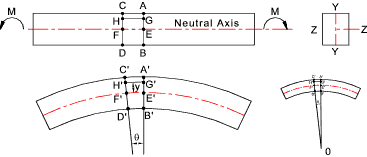Assumptions The beam is symmetrical about Y-Y The traverse plane sections remain plane and normal to the longitudinal fibres after bending (Beroulli's assumption) The fixed relationship between stress and strain (Young's Modulus)for the beam material is the same for tension and compression ( σ= E.e ) Consider two section very close together (AB and CD). After bending the sections will be at A'B' and C'D' and are no longer parallel.   AC will have extended to A'C' and BD will have compressed to B'D' The line EF will be located such that it will not change in length.   This surface is called neutral surface and its intersection with Z_Z is called the neutral axis The development lines of A'B' and C'D' intersect at a point 0 at an angle of θ radians and the radius of E'F' = R Let y be the distance(E'G') of any layer H'G' originally parallel to EF..Then H'G'/E'F' =(R+y)θ /R θ = (R+y)/R And the strain e at layer H'G' = e = (H'G'- HG) / HG = (H'G'- HG) / EF = [(R+y)θ - R θ] /R θ = y /R The accepted relationship between stress and strain is σ= E.e Therefore σ = E.e = E. y /R σ / E = y / R Therefore, for the illustrated example, the tensile stress is directly related to the distance above the neutral axis.   The compressive stress is also directly related to the distance below the neutral axis.   Assuming E is the same for compression and tension the relationship is the same. As the beam is in static equilibrium and is only subject to moments (no vertical shear forces) the forces across the section (AB) are entirely longitudinal and the total compressive forces must balance the total tensile forces.  The internal couple resulting from the sum of ( σ.dA .y) over the whole section must equal the externally applied moment.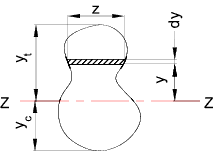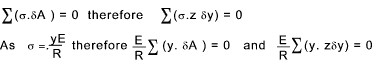This can only be correct if Σ(yδa) or Σ(y.z.δy) is the moment of area of the section about the neutral axis.  This can only be zero if the axis passes through the centre of gravity (centroid) of the section. The internal couple resulting from the sum of ( σ.dA .y) over the whole section must equal the externally applied moment.  Therefore the couple of the force resulting from the stress on each area when totalled over the whole area will equal the applied moment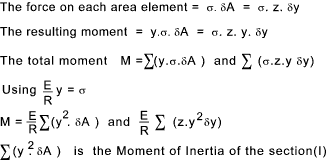From the above the following important simple beam bending relationship results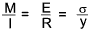It is clear from above that a simple beam subject to bending generates a maximum stress at the surface furthest away from the neutral axis.  For sections symmetrical about Z-Z the maximum compressive and tensile stress is equal. σmax = ymax. M / I The factor I /ymax is given the name section Modulus (Z) and therefore σmax = M / Z Values of Z are provided in the tables showing the properties of standard steel sections Deflection of Beams Below is shown the arc of the neutral axis of a beam subject to bending.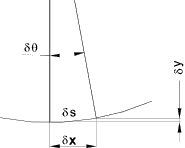For small angle dy/dx = tan θ = θ The curvature of a beam is identified as dθ /ds = 1/R In the figure δθ is small and δx; is practically = δs; i.e ds /dx =1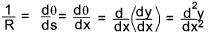From this simple approximation the following relationships are derived.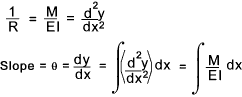Integrating between selected limits. The deflection between limits is obtained by further integration.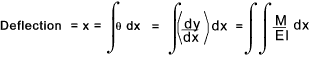It has been proved ref Shear - Bending that dM/dx = S and dS/dx = -w = d2M /dx Where S = the shear force M is the moment and w is the distributed load /unit length of beam.   therefore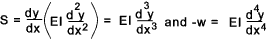If w is constant or a integratatable function of x then this relationship can be used to arrive at general expressions for S, M, dy/dx, or y by progressive integrations with a constant of integration being added at each stage.  The properties of the supports or fixings may be used to determine the constants. (x= 0 - simply supported, dx/dy = 0 fixed end etc ) In a similar manner if an expression for the bending moment is known then the slope and deflection can be obtained at any point x by single and double integration of the relationship and applying suitable constants of integration.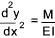Singularity functions can be used for determining the values when the loading a not simple ref Singularity Functions Example - Cantilever beam Consider a cantilever beam (uniform section) with a single concentrated load at the end.  At the fixed end x = 0, dy = 0 , dy/dx = 0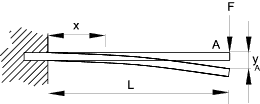From the equilibrium balance ..At the support there is a resisting moment -FL and a vertical upward force F. At any point x along the beam there is a moment F(x - L) = Mx = EI d 2y /dx 2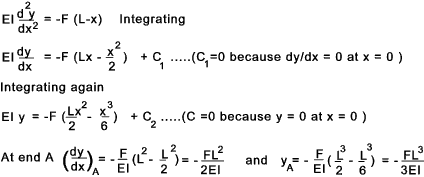Example - Simply supported beam Consider a simply supported uniform section beam with a single load F at the centre.    The beam will be deflect symmetrically about the centre line with 0 slope (dy/dx) at the centre line.   It is convenient to select the origin at the centre line.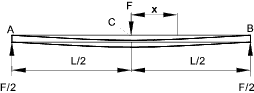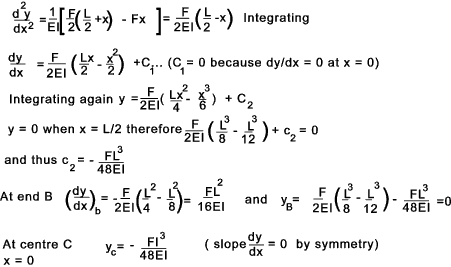Moment Area Method This is a method of determining the change in slope or the deflection between two points on a beam.  It is expressed as two theorems... Theorem 1 If A and B are two points on a beam the change in angle (radians) between the tangent at A and the tangent at B is equal to the area of the bending moment diagram between the points divided by the relevant value of EI (the flexural rigidity constant). Theorem 2 If A and B are two points on a beam the displacement of B relative to the tangent of the beam at A is equal to the moment of the area of the bending moment diagram between A and B about the ordinate through B divided by the relevant value of EI (the flexural rigidity constant). Examples ..Two simple examples are provide below to illustrate these theorems Example 1) Determine the deflection and slope of a cantilever as shown..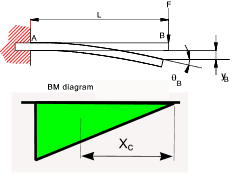The bending moment at A = MA = -FL The area of the bending moment diagram AM = -F.L2 /2 The distance to the centroid of the BM diagram from B= xc = 2L/3 The deflection of B = y b = A M.x c /EI = -F.L 3 /3EI The slope at B relative to the tan at A = θ b =AM /EI = -FL2 /2EI Example 2) Determine the central deflection and end slopes of the simply supported beam as shown.. E = 210 GPa ......I = 834 cm4...... EI = 1,7514. 10 6Nm 2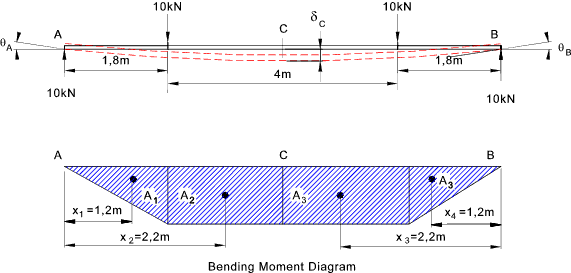A1 = 10 . 1,8 . 1,8 /2 = 16,2kNm2 A2 = 10 . 1,8 . 2 = 36kNm2 A3 = 10 . 1,8 . 2 = 36kNm2 A4 = 10 . 1,8 . 1,8 /2 = 16,2kNm2 x1 = Centroid of A1 = (2/3) . 1,8 = 1,2m x2 = Centroid of A2 = 1,8 + 1 = 2,8m x3 = Centroid of A3 = 1,8 + 1 = 2,8m x4 = Centroid of A4 = (2/3) . 1,8 = 1,2m The slope at A is given by the area of the moment diagram between A and C divided by EI. θA = (A1 + A2) /EI   =   (16,2+36).10 3 / (1,7514. 10 6) =  0,029rads   =   1,7 degrees The deflection at the centre (C) is equal to the deviation of the point A above a line that is tangent to C. Moments must therefore be taken about the deviation line at A. δC = (AM.xM) /EI   =   (A1 x1 +A2 x2) / EI   =   120,24.10 3/ (1,7514. 10 6) =   0,0686m = 68,6mm Composite Beams Beams constructed of more than one material can be treated by using the equivalent width technique if the maximum stresses in each of the materials is within the relevant materials elastic limit.    Consider a composite beam as shown below.    The steel has an elastic modulus ES = 210.103 N/mm2 and the aluminium has an EA = 78.103 N/mm2.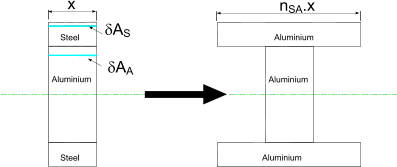The composite beam is analysed on the basic assumption that plane surfaces remain plane during bending within the elastic limit therefore down the full depth of the beam the strain (deflection /original length) is constant i.e the deflection is proportional to the distance from the neutral axis of the beam.    The strain is equal to the (stress /Youngs Modulus(E)) Refer to figure below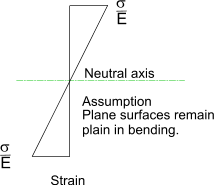Now to obtain the equivalent section which is all aluminium the dimensions of the replacement aluminium must be such that the mechanical properties are equivalent to the original material.    The overall depth of the transformed section is the same as the orginal section. The resulting strain in any element dA of the transformed section must constant.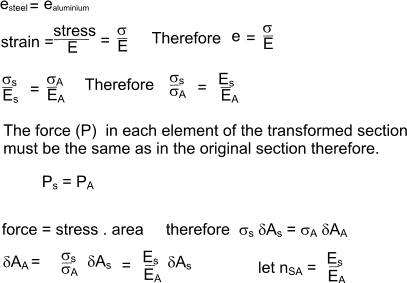The equivalent area of the transformed section based on aluminium is equal to the area of the original steel section x nSA.     If the depth of the transformed section is the same as the original section then the width of the transformed aluminium section is equal to nSA x width of original steel section. The equivalent area of the aluminium section must be subject to the same strain as original steel section positioned at the same distance from the section neutral axis.    The simple beam theory can be used to calculate the bending stresses in the transformed section. The actual stresses will, of course, be n x the calculated stresses in the transformed section. Example on composite beams Consider a composite beam comprising steel, brass, and aluminium sections.     Produce an equivalent section based on Aluminium.    Calculate the position of the neutral axis and the moment of inertia of the equivalent section.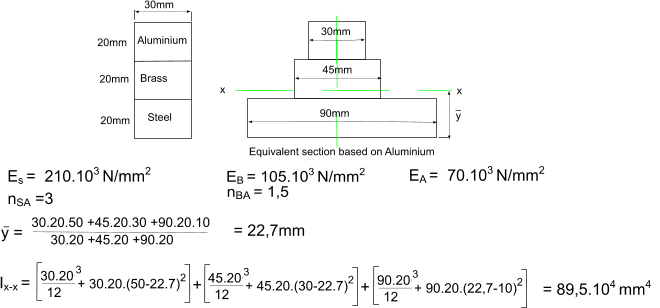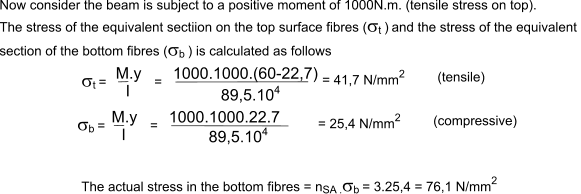Sites Providing Relevant Information Pure Bending...Mississipi State U. Pure Bending Lecture Notes.. very useful simple Bending theory...Very useful set of Notes Introduction to beam theory...Very simple easy to follow notes web.clark.edu/bwheeler/Engr%20233/.../Bending%206.3-6.4.ppt
Beams Index